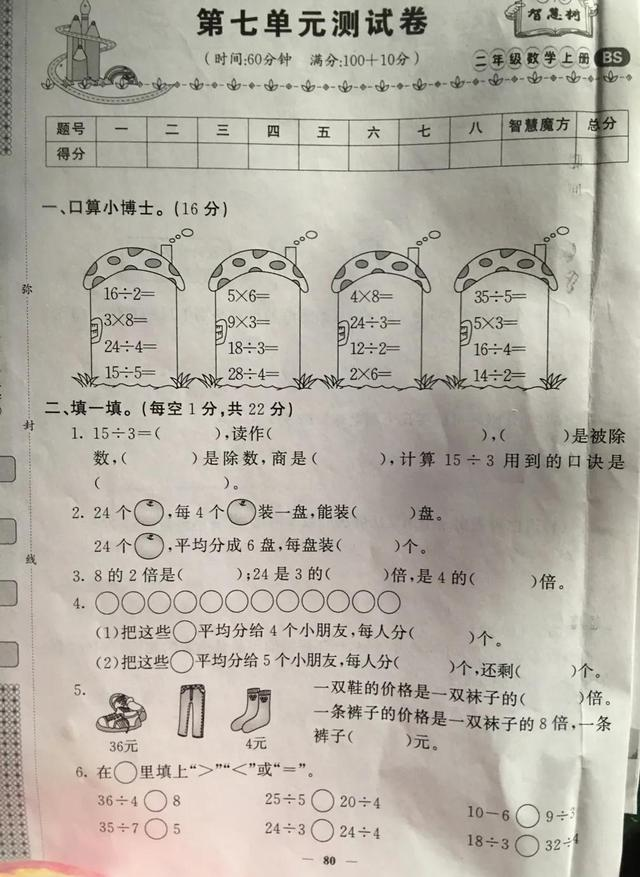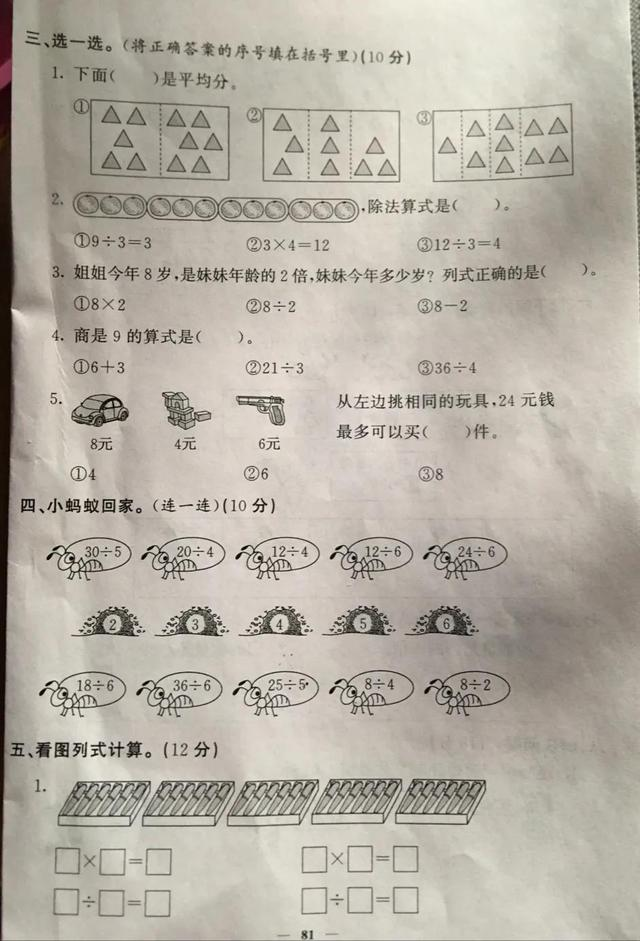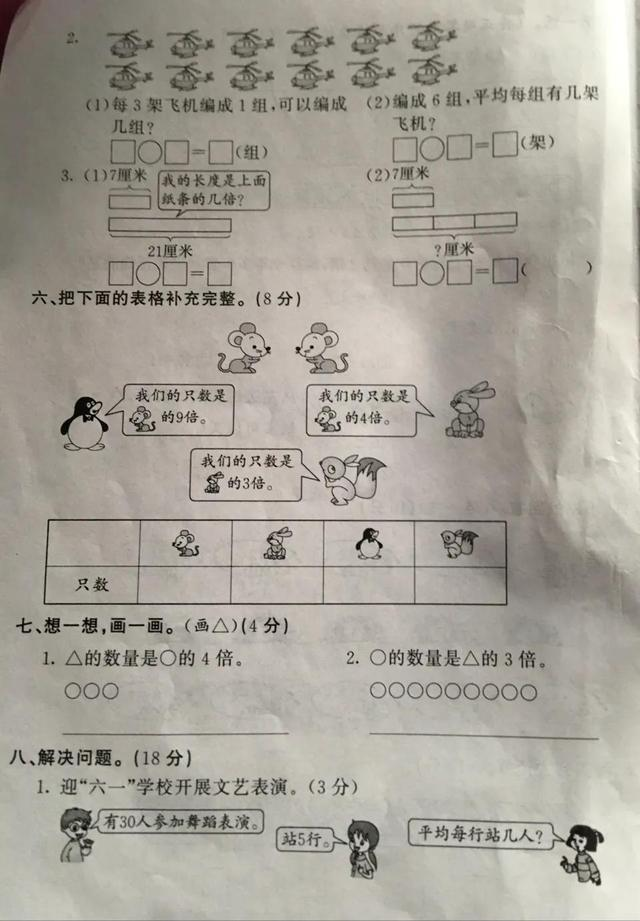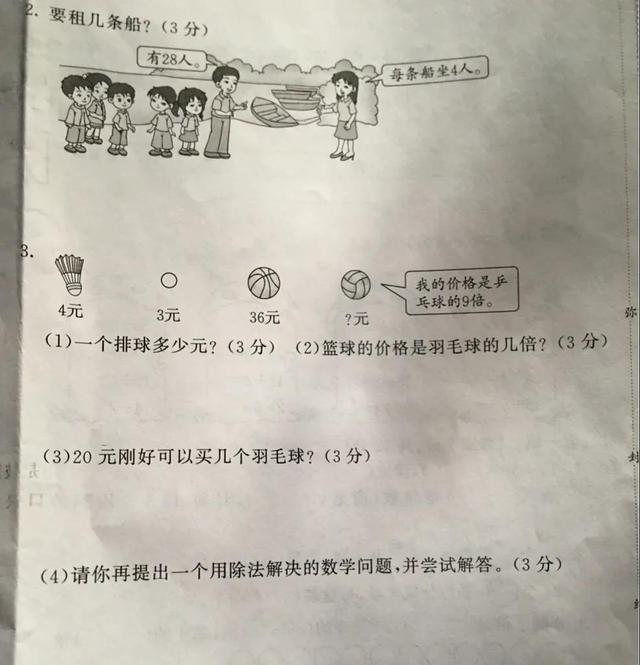# 北师大版二年级上册数学第七单元《分一分与除法》测试卷，请收藏

2021-01-04 63 ℃16÷2=8，5×6=30，4×8=32，35÷5=7，

3×8=24，9×3=27，24÷3=8，5×3=15，

24÷4=6，18÷3=6，12÷2=6，16÷4=4，

15÷5=3，28÷4=7，2×6=12，14÷2=7。20元可以买几个羽毛球？20÷4=5个。